## What is Internal Compound Stability?

Retaining wall designs have typically been limited to internal stability, external stability and bearing analysis by the site civil engineer or the wall design engineer. Additionally, the overall stability of the site is the responsibility of the owner and should be addressed by the owner, by contracting with a geotechnical engineering firm. The geotechnical engineering firm should provide a full global analysis of the entire site including the effects of the segmental retaining walls.

As the design roles become more defined it has become more customary for an Internal Compound Stability (ICS) analysis to be performed. ICS calculations determine the factors of safety for potential slip surfaces which pass through the unreinforced retained soil, the reinforced soil mass and the wall facing within the wall design envelope.Figure 1 Internal Compound Analysis

Internal compound stability calculations are limited to a wall design envelope above the base material and back no further than 2 (H) or He + L, whichever is greater. This evaluation zone models the slip surface through the wall facing. The slip surface slices the affected grid layers and shears or bulges the SRW facing units. The designers performing ICS calculations can now model the entire wall design envelope in one comprehensive calculation. These calculations include the effects of the infill and retained soil strength, the individual grid layer strengths and spacing and the shear and connection strength the SRW facing brings to the system.

The distinctions between an ICS analysis and a global stability analysis form a clear line of design responsibility. A site civil or wall designer should review the ICS above the base material and through the wall facing within the design envelop for each wall designed on a site. For the larger site stability design, the owner through their geotechnical engineer should be responsible for the global stability of the entire site including the soils below the base material of all walls and structures designed on the project site.

## Design MethodologyFigure 2 Internal Compound Stability Design Envelope

The Simplified Bishop Method of Slices (see References) is one of the most common analysis methods used in global stability modeling of reinforced slopes. In this method the volume, or weight, of the soil above a slip surface is divided into vertical wedges. The weight of soil is used to calculate the forward sliding forces as well as the sliding resistance due to the frictional interaction with the soil along the slip surface. In the ICS calculations we use the same process of evaluating the soil interaction, but additionally, the ICS analysis combines the resisting forces developed by geogrid layers intersecting the slip arc and the contribution from the SRW facing. Current slope stability modeling either ignores the facing or tries to mimic it by exaggerating a thin semi-vertical soil layer. Internal compound stability calculations analyze both the facing shear capacity and the facing connection capacities to formulate a reasonable facing contribution to the resistance side of the equation. By combining these multiple sliding and resisting forces along the slip surface, a safety factor equation is formed by a ratio of resisting forces to the sliding forces. The end result determines if there is an equilibrium of forces along a particular slip surface

The following equation calculates the Factor of Safety of Internal Compound Stability:

### Safety Factor of ICS

= (∑ Fr + ∑ Fgr)/(∑ Fs + ∑ Fdyn)

 Where: ∑ Fr = sum of soil resisting forces ∑ Facing = sum of facing contribution ∑ Fgr = sum of geogrid contribution ∑ Fs = sum of sliding force ∑ Fdyn = sum of sliding forces due to seismic loading

## Soil Sliding and Resting Forces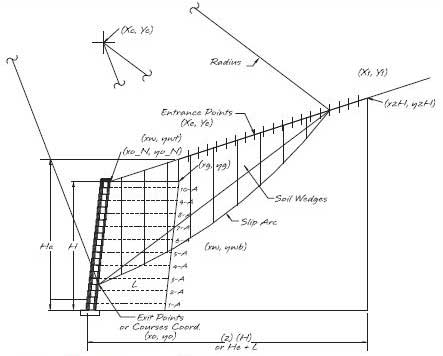Figure 3: Internal Compound Stability Diagram

As mentioned earlier, the Simplified Bishop Method of Slices is used to determine first the weight of the soil above the slip surface and then the sliding and resisting forces due to that soil weight along the slip surface. Figure 3 shows a typical section through the evaluation zone for ICS calculations. The vertical slices in the soil above the slip arc represent the individual portions of soil analyzed using Bishops theory. We will determine the weights and forces relative to one soil slice or wedge as an example. For a complete Simplified Bishop Method of Slices the designer would follow the same calculations for each individual soil wedge and at the end, sum them all together

In Bishop modeling the soil wedges can be calculated as individual parts due mainly to Bishop's assumption that the vertical frictional forces between soil wedges are neglected, meaning that for design purposes there is no interaction between individual soil wedges. Therefore, the individual soil wedge weight (W) is determined simply by multiplying the volume of soil in that wedge by the unit weight of the soil. To determine the individual wedge volumes the designer must determine the exact geometry of the wall section and the slip arc to be evaluated. This is complex geometry that varies for every slip arc so it is a very difficult calculation to perform by hand. Please note that the thinner the wedge slice is the less the loss of weight is in the calculations. That is, the bottom of each wedge is considered a straight chord, not an arc, for ease of calculations. The lost soil weight is the area below the bottom chord and arc, and is negligible when the wedges are thinner.

Once the wedge weight is determined the forward sliding force (Fs) is calculated by multiplying it by the sine of the angle below the soil wedge (α), where α is defined as the angle between horizontal and the bottom chord of each soil wedge; α is different for each wedge due the relative location of each wedge along the slip surface.Figure 4: Lost Soil Weight

Sliding Force:

Fs = (Weight Wedge) sin (α)

Compare for a moment two wedges, W1 = 1000 lb/ft (14.6 kN/m) and W2 = 100 lb/ft (1.46 kN/m). The first (W1) is near the bottom of the slip arc where the arc ends near the facing and is relatively flat and therefore the angle is relatively small, say 10 degrees. The other (W2) is near the top of the slip arc where the arc is steeper and therefore the α angle is steeper, say 60 degrees. The sine (α) term acts as a percentage of forward movement, i.e. the flatter the α angle the smaller percentage:

• FS1
• = (W1) sin (10 degrees) = 1000 lb/ft (0.174)
17.4% of (1000 lb/ft) = 174 lb/ft (2.54 kN/m)
• FS2
• = (W2) sin (60 degrees) = 100 lb/ft (0.866)
86.6% of (100 lb/ft) = 86.6 lb/ft (1.26 kN/m)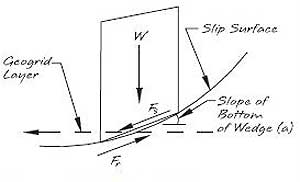Figure 5: Wedge Force Diagram

The sliding resisting force (Fr) is calculated by multiplying the wedge weight by tangent of the internal friction angle of soil, which is commonly used for the soil frictional interaction coefficient. However, Bishop's method then divides this term by a geometric equation called mα; mα is a relationship between the strength of the soil and the relative angle of slip (α) for each wedge and is moreclearly defined in global stability text books or global stability modeling programs such as ReSSa.

Sliding Resisting Force(Fr):

Fr = (Weight Wedge) tan (Φ)/mα

Where:

mα = cos (α) + [sin (α) tan (Φ)] / FSi

And FSi is the initial safety factor used to start the iteration process.Figure 6: Wedge Weight"

Generally, the Simplified Bishop procedure is more accurate than the Ordinary Method of Slices, but it does require an iterative, trial-and-error solution for the safety factor. Therefore, the designer needs to approximate what the safety factor will be for the final resulting slip surface. The closer the initial approximation is to the actual safety factor, the less iteration that will be required. This iteration process is standard for a Bishops calculation and again stresses the point that it is difficult to do hand calculations

### Surcharges and Seismic Forces

Surcharge and seismic forces are calculated very similarly in a Bishops model.Surcharges, whether live or dead are simply added to the weights of the individual soil wedges. It should be noted that in an ICS calculations there is no distinction between live and dead load. By handling it in this manner the wedge weight term is increased by the relative weight of the surcharge and is than carried through the Sliding Force (Fs) and the Sliding Resisting Force (Fr) calculations. The designer should be careful to analyze where the surcharges are applied so they add that weight to only the effected soil wedges.

Therefore, the Sliding Forces and Sliding Resisting Force equations are redefined as:

• Sliding Force
• Fs= (Weight Wedge + Weight Surcharge) sin(α)
• Sliding Resisiting Force
• Fr= (Weight Wedge + Weight Surcharge) tan (Φ) / mα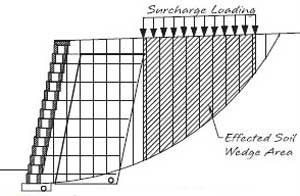Figure 7: Effect of Surcharge Loading

The Seismic Force (Fdyn) for a particular slip surface is additive to the Sliding Force (Fs) and is calculated by multiplying Fs by the horizontal acceleration coefficient (kh); kh is defined in Chapter 5, Seismic Analysis.

Fdyn = (Fs)(kh) or for all wedges:  ∑ Fdyn =  ∑ Fs(kh)

### Geogrid Contribution (Fgr):

It would stand to reason that if a layer of geogrid is passed though by a slip arc, that the geogrid strength would increase the safety factor or stability of that slip surface. Therefore the relative geogrid interaction (Fgr) will be directly added to the resisting side of the equilibrium equation. The grid interaction in this calculation is directly effected by the geogrid spacing. If grid layers are closer together there is a higher likelihood of grid layers being passed through by the slip surface, thus providing more geogrid interaction. The greater the grid spacing the greater possibility of the slip surface falling between grid layers and thus not increasing the slip surfaces stability.Figure 8: Geogrid Conribution at the Slip Arc

The horizontal resistance forces due to geogrid layers that intersect the slip arc are determined by the lesser of either the pullout of soil strength or the long term allowable load strength (LTADS) of the geogrid. Both are defined in the Internal Stability section of Chapter 2. The pullout of soil is calculated by determining the embedment length (Le) on either side of the slip surface and combining it with the confining pressure, or normal load, from the soil above.

The designer should consider that there are two sides of the slip arc to consider when calculating the geogrid contribution. If the slip arc breaks free from the soil resistance along the slip surface, it will engage the affected geogrid layers. The grid layers can fail in three ways. First the grid can be pulled out from the soil on the retained side of the slip surface. Second, the geogrid layer can be pulled out from the soil on the sliding side of the slip surface. But on this side, the designer must take into account that the end of the grid is connected to the facing. Therefore the total pullout strength on the sliding wedge side is the connection strength plus the pullout of soil. This is a very unlikely way for the grid to fail because this combination will most always be greater than the rupture strength of the grid (limited to the LTADS). Third, the grid can rupture if the pullout of soil strengths exceeds the LTADS of any affected layer.Figure 9: Grid Force

Calculations show that it is most likely that if a slip occurs some layers will pullout from the retained side and at the same time some layers will rupture.

The designer should analysis each layer of effected geogrid for the three failure modes to determine the lesser for each layer, and then the sum of these lesser amounts becomes the  ∑ Fgr value.

### Wall Facing Contribution (Facing):

One element of the ICS calculations is the inclusion of facial stability to add to the sliding resistance. The stability of the wall facing has typically been ignored in global modeling due to the complexity of modeling a segmental retaining wall into a slope stability computer program.

Wall facing stability is provided by the interlocking shear between block and by the connection capacity between block and geogrid. Both are directly related to the spacing of the geogrid layers and the amount of normal load above the area in question. The closer together the reinforcement layers are, the more stable the facing becomes in both shear and connection strength. The maximum spacing between grid layers that can be found within the industry is around 32 in. (812 mm). However, past experience has shown that retaining walls that have geogrid layers spaced too far apart do not yield the best design for a wall. Problems associated with excess settlement, deflection and bulging may be experienced. Allan Block recommends a geogrid spacing of 16 in. (406 mm) or less. Closer spacing of lower strength reinforcement is a more efficient way of distributing the loads throughout the mass, which creates a more coherent structure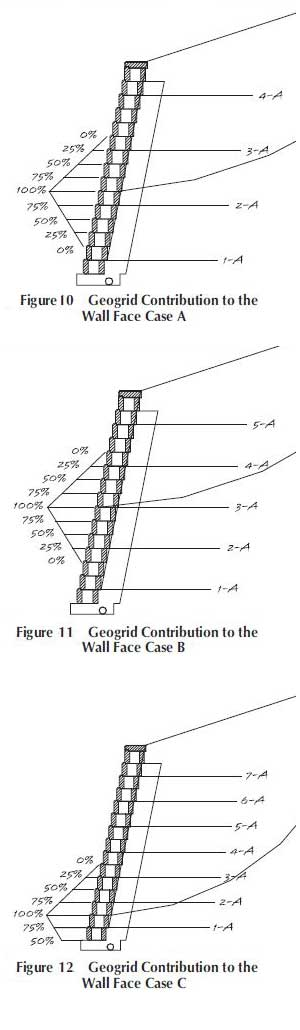Please note that the designer must evaluate both the stability provided by the geogrid connection and the shear strength of the block units, but can only use the lesser of the two in the ICS safety factor equation. Understanding that these two stabilizing forces are interconnected is a benefit to the designer of reinforced segmental retaining walls.

### Facing Stability from Geogrid Connections

In the internal compound stability analysis, when the slip arc travels through the wall face at a grid layer we can safely assume that the full connection capacity is available to resist the sliding. However, the grid layers at the face that are above and below the slip arc will also provide some resistance and increase stability. Using a maximum influence distance of 32 in. (812 mm) from the slip arc, a percentage of the grid connection is used in calculating the contribution from block to grid connections when evaluating facial stability. Here are a few examples showing different spacing and slip arc locations.

In Case 1 the slip arc is directly above a layer of geogrid and there are two layers that fall within the influence zone of 32 in. (812 mm) on either side of the slip arc. Looking at how the percentages are distributed, 75% of Grid 2A and 25% of Grid 3A connection strength capacities can be in the analysis of the wall facing. Assuming a full 8 in. (200 mm) tall unit.

Case 2 has three course spacing between grids and the slip arc intersecting the wall face at a geogrid layer. Therefore 100% of Grid 3A and 25% of Grids 2A and 4A connection strength capacities can be included.

Case 3 illustrates the boundary layers. The slip arc is towards the bottom of the wall, which means the bottom portion of the influence zone actually includes the bottom of the wall. Grid connection strength capacities are easily identified at 25% of Grid 3A and 75% of Grids 1A and 2A. However, because the slip arc is located towards the bottom of the wall we can also include 50% of the frictional sliding resistance between the Allan Block unit and the gravel base.

### Facing Stability from Block Shear Strength

Shear interaction between units is easily calculated by understanding that the greater the normal load above a particular joint, the greater the block-to-block shear strength becomes. The tested shear strength equation comes from each SRW manufacture in the form of an ASTM D6916 test (also known as SRW-2 and is included in the appendices), which determines the block-grid-block shear resistance and block-block shear resistance relative to the normal load above that joint.

The first thing a designer should do is determine if the slip surface in question passes through the facing at a geogrid layer. If it does the assumption is made that the facing is 100% stable due to the connection strength with the geogrid and thus the designer can consider adding the tested block-grid-block shear strength of that joint in the analysis of the wall facing.

If the slip surface passes through the facing between grid layers a rotational moment develops between grid layers, with the lower grid layer forming a pivot point for the potential wall facing bulge. Summing the moments about this pivot point the designer can determine if the normal load at that joint is substantial enough to resist the upward rotational effect caused by the sliding forces. If there is sufficient normal load to resist the rotational effect the block will not uplift and the designer can consider adding the full block-block shear strength into the sliding resistance. However, if the normal load is overcome by the rotational uplift, the wall facing will pivot forward and the shear strength of the block cannot be added to the resistance.

Ultimately, this forward rotation will engage the geogrid connection strength from the grid layer above which will act to restrain the facing. If the wall continues to rotate, more uplift will occur and a forward bulge will form between layers and eventually a localized wall failure will occur.

### Contribution from the Wall Face

As mentioned earlier, the designer cannot take both the facing stability from the geogrid connection and block shear when totaling up the resisting force. Only one will need to fail before instability of the wall face occurs. Therefore, the one with the least resisting force is the controlling face contribution and is used in the ICS safety factor calculation. The basis of this approach relies on a simple theory that as reinforcement layers are placed closer together, the facing becomes more rigid. The more rigid the facing is made by the connection contribution, the more likely that the shear strength at the evaluated course will control. Likewise, as the geogrid spacing is increased, the connection contribution is lessened thus causing the connection contribution to control.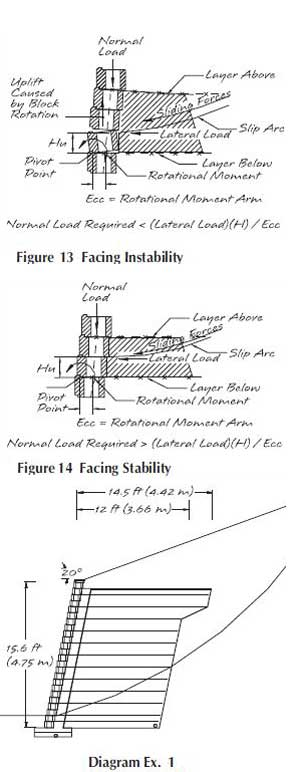The following is an example of evaluating ICS for a give set of site and soil conditions. Please note that a full global stability review should be obtained by the owner. These types of calculations require hundreds of thousands of iterations, while evaluating tens of thousands of slip arcs.

Example 6-1:

Looking at Diagram Ex. 6-1 and given the following:

 β = 78° γ = 120 lb/ft3(19 kN/m3) Φi = 30° Αο= 0.25 Φr = 28°

Geogrid is spaced 2 courses apart and a minimum length of 12 ft (3.66 m).
The LTADS for this example is approximately 1,008 lb/ft (14.7 kN/m).

Reviewing the full ICS analysis, it is determined that the minimum Factor of Safety for ICS occurs between the 2nd and 3rd course of blocks.

The following summarizes the results for the slip arc with the minimum Factor of Safety for ICS:

∑ Fr    = sum of soil resisting forces     = 18,156 lb/ft (265 kN/n)

∑ Facing= sum of facing contribution (either geogrid connection or shear)

∑ Vu     = sum of block shear     = 4,082 lb/ft (59.6 kN/m)

∑ Conn     = sum of connection     = 4,819 lb/ft (70.4 kN/m)

∑ Facing     = 4,082 lb/ft (minimum of the shear and connection) (59.6 kN/m)

∑ Fgr     = sum of geogrid contribution     = 2,791 lb/ft (40.7 kN/m)

∑ Fs    = sum of sliding force     = 17,608 lb/ft (257kN/m)

∑ Fdyn     = sum of sliding forces due to seismic loading     = 1,585 lb/ft (23.1 kN/m)

Safety Factor of ICS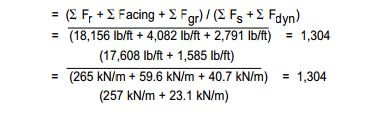### Safety Factors and Design Approach

The minimum safety factor for Internal Compound Stability is 1.3 for static conditions and 1.1 for seismic. If after completing the analysis the safety factors are below these standards, the wall design will need to be revised. Please note that to provide a conservative expanded review for a geogrid reinforced retaining wall when analyzing ICS, cohesion is not considered in the methodology presented. Most global stability computer programs provide for the engineer to include a value for cohesion, which would dramatically change the final numbers. Additionally most global stability programs have not provided a detailed approach to contributions from the wall facing and therefore the exact results will be difficult to duplicate when trying to run a comparative review with off the shelf GS software. The following provides a few design options to increase factors of safety for Internal Compound Stability:ICS Force Summary

1. Use select backfill: It has been well documented that using select soils with higher internal strength as backfill in the infill area results in a better wall with increased stability and performance. This will also improve the internal compound stability as well and should be one of the first recommendations.

2. Additional geogrid reinforcement layers: Decreasing the spacing between the geogrid reinforcement will force the slip surface to intersect more geogrid layers which will increase the safety factor. The wall facing stability will also improve and will have a direct enhancement in the internal compound stability analysis.

3. Lengthen the geogrid reinforcement: Lengthening the geogrid will, again, force the slip surface to intersect more layers of geogrid and ultimately force the slip surface deeper into the evaluation zone. However, this will require additional excavation, and out of the three design options will typically cost the most.

4. Addition of geogrid in the slope above the wall: For slopes above the wall, adding geogrid reinforcement within the slope may improve Internal Compound Stability. The length and spacing of these grids will depend on the site conditions and should be done in cooperation with the geotechnical engineer of record.